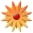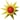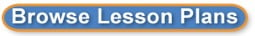# A Matter of Fact

Page Views: 6459

Email This Lesson Plan to Me
Email Address:
Subscribe to Newsletter?
Log in to rate this plan!
Overall Rating:(5.0 stars, 2 ratings)

 Keywords: Math, Science, Matter, Technology Subject(s): Grades 5 through 6 School: Pembroke Pines Elem School, Pmbk Pines, FL Planned By: C A Original Author: christine andreu, Pmbk Pines
this unit of instruction began with a week long lesson on the system of units that make up all matter and the properties of matter. Students were introduced to the building blocks of matter and how matter can be compared and measured. The scientific and mathematical tool of a balance scale was introduced and students were able to measure the masses of various classroom objects using the metric system and precision of measurement. Students incorporated the mathematical skills of dividing, multiplying and comparing decimals to compare mass and weight and discussed how these differ from one another. Through the use of an online video clip students saw a demonstration on how their mass would stay the same on the moon, but their weight would change due to the change in gravity. They also learned that the weight of an object always equals the sum of its parts. This was done during a math lesson in which a scale was used to measure the weight of connected cubes and compared to the sum of the weights for each cube measured individually.
During the second week of the unit students were introduced to the concept of volume and how volume can be measured. Students experienced measuring the volume of a liquid using a graduated cylinder and also measured the volume of a solid through displacement. Finally, students participated in small groups on an experiment involving the affect of pressure on the volume of a gas using syringes and measuring the volume of the gas in milliliters. To aid students in grasping the concept of volume, they calculated the volume of various containers using the mathematical formula for volume to provide a more hands on experience. This provided an opportunity for students to connect their understanding of volume to previous knowledge of capacity that they had learned in mathematics. At the end of the activity students created a data analysis chart and constructed a line graph to represent their data in mathematics.
During the third and fourth week of the unit students were given an opportunity to connect their understanding of mass and volume as they began to learn about density as the mass per unit of volume. After learning what density was and the appropriate formula for figuring the density of an item, students participated in small groups to complete several activities in which they utilized balance scales to calculate the mass of solids and liquids. They also used graduated cylinders to measure the volume of these items. Then they used the mathematical formula to determine the density of various solids and liquids and compared the densities to determine which was the most or least dense by dividing decimals. Finally, they used wooden dowels and metric rulers to perform float tests on different liquids and compared the measurements in fractions using the float height to relate floating to the density of a liquid. The data that they gathered in a table was used to create a bar graph on a graphing web site.
In the fifth and sixth weeks of the unit students experimented in their groups with the properties of matter specifically, solubility as they worked with solutions and suspensions. They used the methods of filtration and evaporation to separate the substances of a mixture and learned that different materials are created by combining substances. During the lesson on atoms and molecules students were introduced to the building blocks of matter and created 3D models of a neutral atom and of a molecule. Through these activities, students were introduced to the periodic table of elements and that matter is made up of parts that are too small to be seen without magnification. Students used algebraic thinking and previous knowledge from our algebra unit in mathematics to write balanced chemical equations for elements and illustrated the bonds that can form an element.
The culminating activity of the unit involved students designing their own science experiments to test a hypothesis related to matter. Students were divided into mixed ability groups and were given the freedom to choose a topic related to one of the concepts taught during the unit. This freedom to choose a topic, provided them with a sense of ownership that gifted and high achieving students seek. They then researched the topic, developed a hypothesis and designed an experiment to test their hypothesis. After completing the experiment each group analyzed their data and activated prior mathematical knowledge to determine what form of graph they should use to represent this data. They then created the graph online using a graphing web site. Based on this data students developed a conclusion and explained how the information that they found could be applied to the world around them. These experiments were developed into science fair projects and students presented their results to peers and family members at the science fair night. Students learned that by using the science process skills they can solve problems, make decisions and form new ideas while parents were able to participate in their childs educational process.
 Materials: Point and Shoot, Portable, Elementary, Video Tools, Printers, Flash/USB Drives